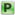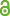Hauptmenü
• Autor
• Bolognini, Davide
• Fugacci, Ulderico
• TitelBetti splitting from a topological point of view
• Datei
• Persistent Identifier
• Erschienen inJournal of Algebra and its Applications
• Erscheinungsjahr2018
• LicenceCC-BY
• Zugriffsrechte• Abstract A Betti splitting $$I=J+K$$ of a monomial ideal $$I$$ ensures the recovery of the graded Betti numbers of $$I$$ starting from those of $$J,K$$ and $$J \cap K$$. In this paper, we introduce this condition for simplicial complexes, and, by using Alexander duality, we prove that it is equivalent to a recursive splitting conditions on links of some vertices. The adopted point of view enables for relating the existence of a Betti splitting for a simplicial complex $$\Delta$$ to the topological properties of $$\Delta$$. Among other results, we prove that orientability for a manifold without boundary is equivalent to admit a Betti splitting induced by the removal of a single facet. Taking advantage of this topological approach, we provide the first example in literature admitting Betti splitting but with characteristic-dependent resolution. Moreover, we introduce the notion of splitting probability, useful to deal with results concerning existence of Betti splitting.